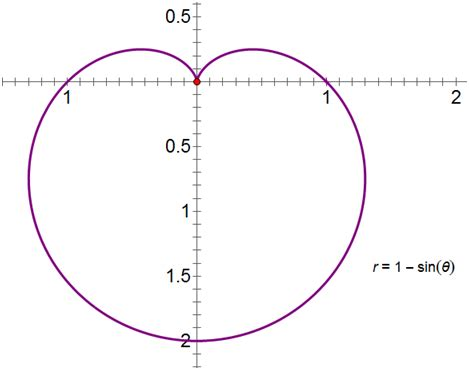💟笛卡尔心形曲线💟Akun 10月 26,2021

笛卡尔心形曲线Matplotlib

Matplotlib 可能是 Python 2D-绘图领域使用最广泛的套件。

作图

笛卡尔心形曲线（代码中有对应解释）

# 因为需要一些专业的数学表达式，所以我们导入numpy
import numpy as np
# 导入matplotlib包
from matplotlib.pyplot import *

# 主函数
if __name__ == "__main__":
# 规定了定义域的范围
t = np.linspace(0, 2 * np.pi, 1000, endpoint=True)
# 规定了x关于t的函数
x = 2 * np.sin(t) - np.sin(2 * t)
# 归定了y关于t的函数
y = 2 * np.cos(t) - np.cos(2 * t)
# 画出y关于x的函数的曲线
plot(x, y, color="red")
# 显示图片
show()众多其它心形曲线

1. 心形公式一，如下。import numpy as np
from matplotlib.pyplot import *

if __name__ == "__main__":
x = np.linspace(-4, 4, 1000, endpoint=True)
y = ((x ** 2) ** (1 / 3) + ((x ** 4) ** (1 / 3) - 4 * (x ** 2) + 4) ** 0.5) / 2
plot(x3, y3, color="yellow")
y = ((x ** 2) ** (1 / 3) - ((x ** 4) ** (1 / 3) - 4 * (x ** 2) + 4) ** 0.5) / 2
plot(x, y, color="yellow")
show()1. 心形公式二，如下。代码如下。

import numpy as np
from matplotlib.pyplot import *

if __name__ == "__main__":
t = np.linspace(-np.pi, np.pi, 1000, endpoint=True)
x = 16 * (np.sin(t) ** 3)
y = 13 * np.cos(t) - 5 * np.cos(2 * t) - 2 * np.cos(3 * t) - np.cos(4 * t)
plot(x, y, color="blue")
show()

运行结果如下图。2. 心形公式三，如下。代码如下图。

import numpy as np
from matplotlib.pyplot import *

if __name__ == "__main__":
x4 = np.linspace(-4, 4, 1000, endpoint=True)
y4 = (2 * np.abs(x4) - x4 ** 2) ** 0.5
plot(x4, y4, color="purple")
g4 = -2.14 * (2**0.5 - np.abs(x4)**0.5) ** 0.5
plot(x4, g4, color="purple")
show()

运行结果如下图（这个公式也报bug，但是~嘿嘿，咱不修，能用就成）。综上

（好久没写过诗了，纯打油，只图能够博得大家一乐）。